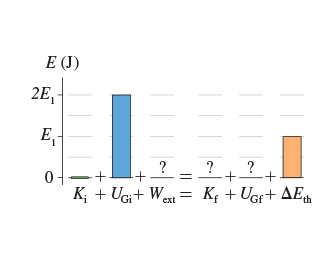# Problem: is the energy bar chart for a firefighter sliding down a fire pole from the second floor to the ground. Let the system consist of the firefighter, the pole, and the earth. Suppose E1 = 14.What is the bar height of Wext?What is the bar height of pandoc: Error at "source" (line 1, column 17): unexpected "{" expecting letter or new-line K_{ m f hspace{1 pt}}?What is the bar height of UGf?

⚠️Our tutors found the solution shown to be helpful for the problem you're searching for. We don't have the exact solution yet.

###### Problem Detailsis the energy bar chart for a firefighter sliding down a fire pole from the second floor to the ground. Let the system consist of the firefighter, the pole, and the earth. Suppose E1 = 14.

What is the bar height of Wext?

What is the bar height of pandoc: Error at "source" (line 1, column 17): unexpected "{" expecting letter or new-line K_{ m f hspace{1 pt}}?

What is the bar height of UGf?